For a Concave mirror, object can be kept at different positions

Hence, we take different cases

### Case 1 - Object is Placed at infinity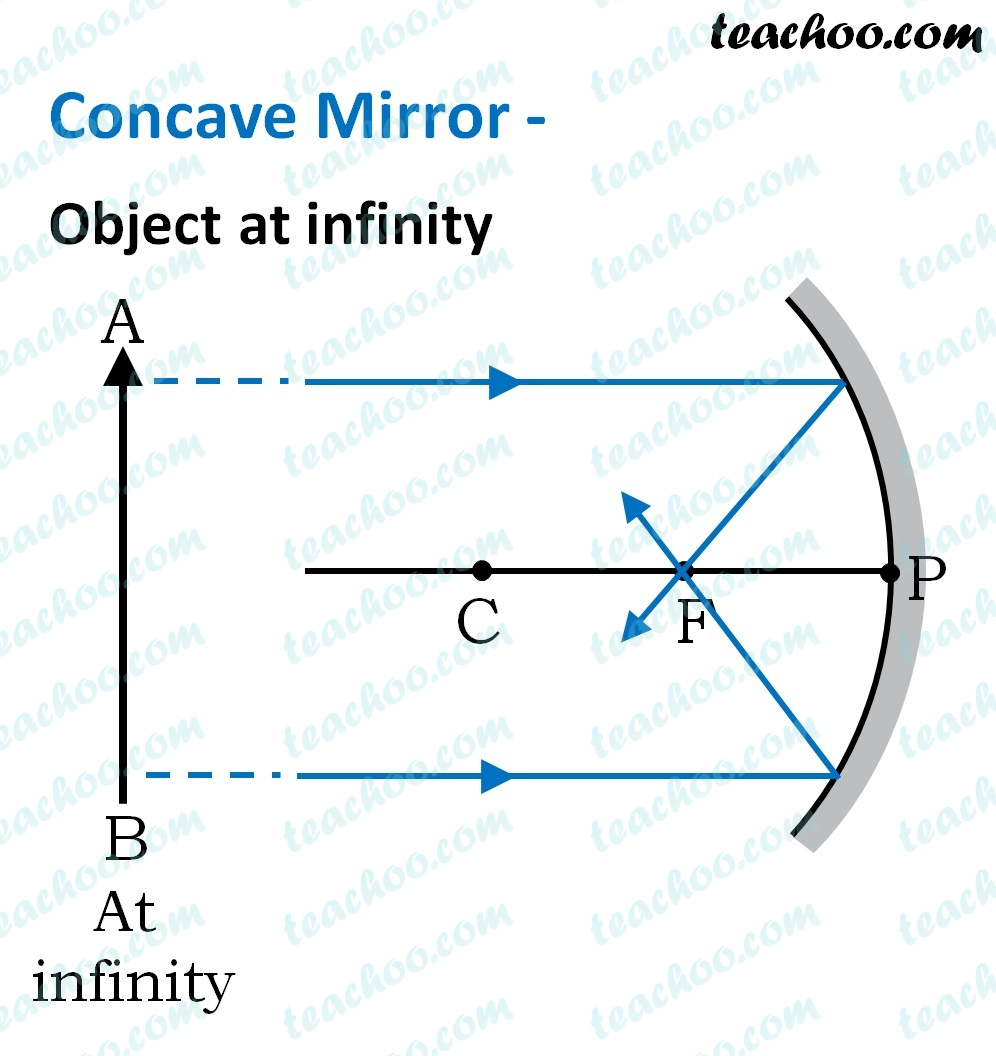In this Case, Object AB is kept far away from mirror (almost at infinite distance)

So, we draw rays parallel to principal axis

Since ray parallel to principal axis passes through the Focus

Both rays meet at focus after reflection

Hence, Image is formed at Focus

And it is very very small

We can say that

• Image is in Front of the Mirror (Real Image)
• Image is Inverted
• Image is Smaller than the Object (Highly Diminished)

### Case 2 - Object is beyond Center of Curvature (C)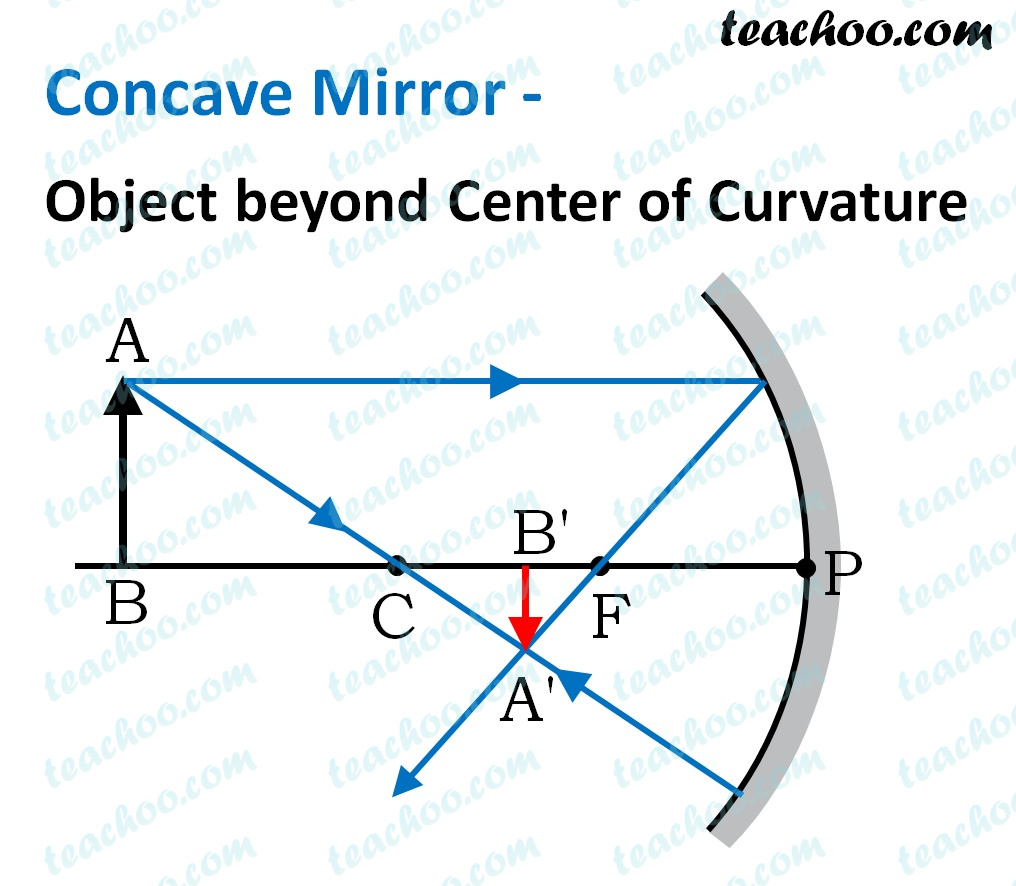Here, Object AB is beyond C

First, we draw a ray parallel to principal axis

So, it passes through focus after reflection

We draw another ray which passes through Center of Curvature

So, the ray will go back along the same path after reflection

Where both reflected rays meet is point A'

And the image formed is A'B'

This image is formed between Center(C) and Focus (F)

We can say that

• Image is in Front of the Mirror (Real Image)
• Image is Inverted
• Image is Smaller than the Object (Diminished)

### Case 3 - Object is at Center of Curvature (C)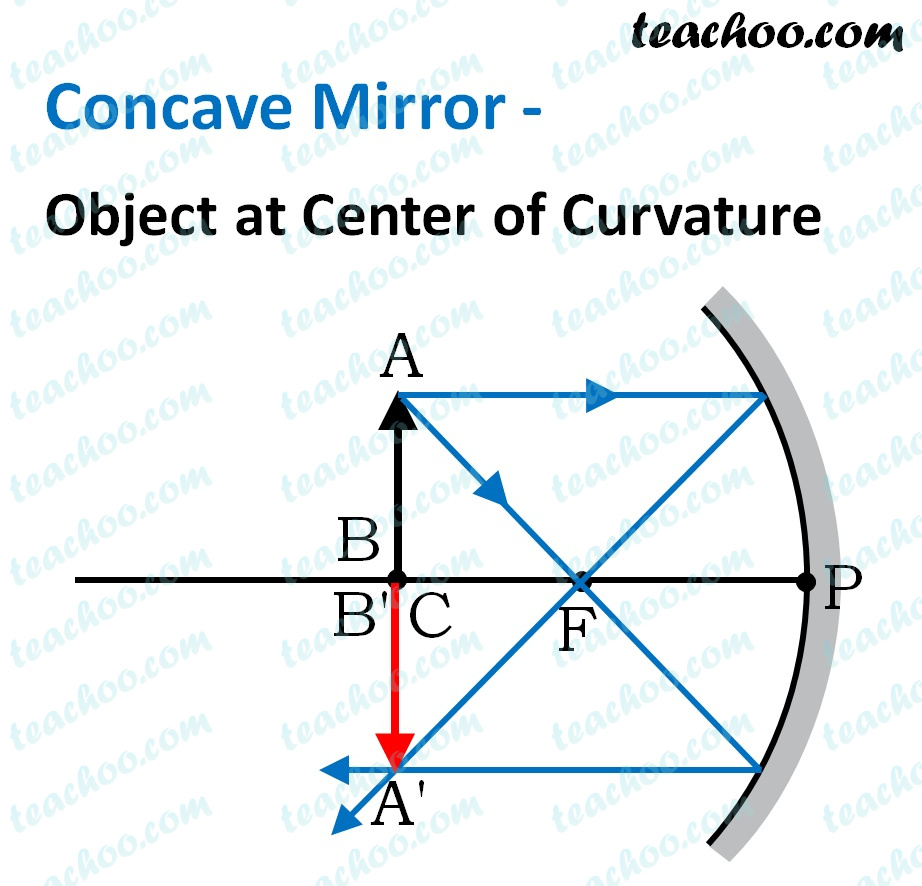Here, Object AB is kept at C

First, we draw a ray parallel to principal axis

So, it passes through focus after reflection

We draw another ray which passes through Focus

So, the ray will reflect back parallel to the principal axis

Where both reflected rays meet is point A'

And the image formed is A'B'

This image is formed between at Center(C)

We can say that

• Image is in Front of the Mirror (Real Image)
• Image is Inverted
• Image is exactly the same size as that of Object

### Case 4 - Object is between Center of Curvature (C) and Focus (F)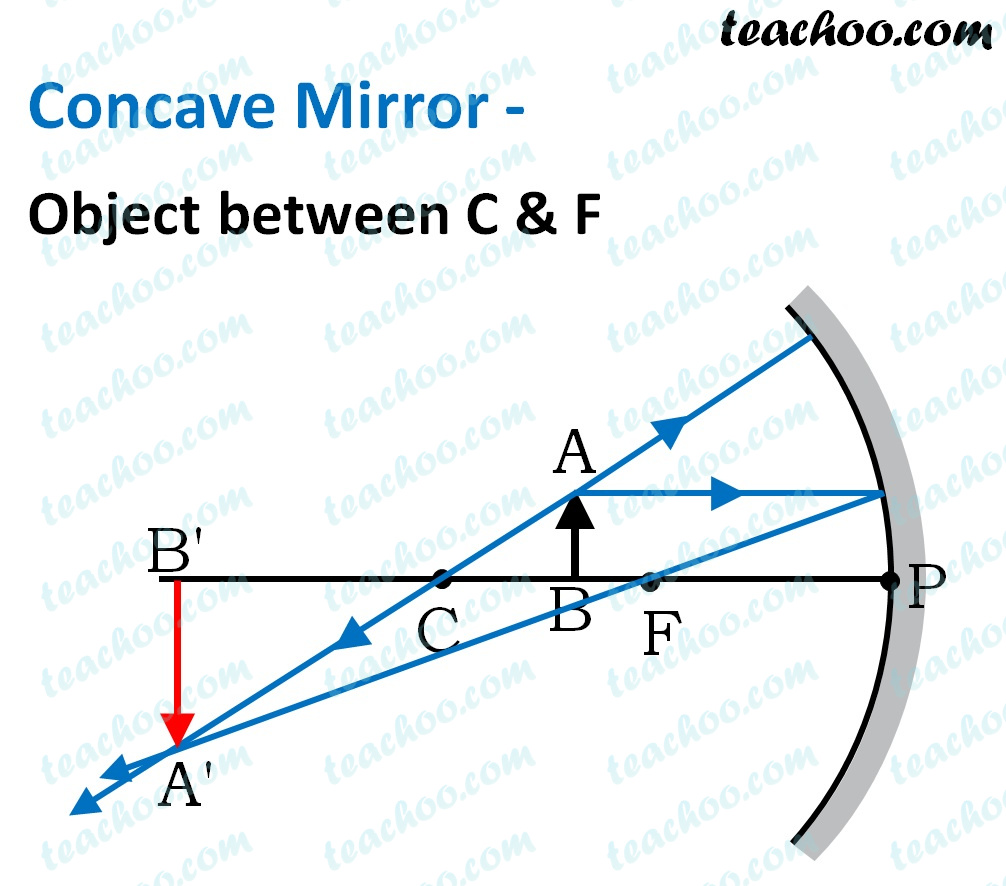Here, Object AB is kept between C and F

First, we draw a ray parallel to principal axis

So, it passes through focus after reflection

We draw another ray which passes through Center of Curvature

So, the ray will go back along the same path after reflection

Where both reflected rays meet is point A'

And the image formed is A'B'

This image is formed between beyond Center(C)

We can say that

• Image is in Front of the Mirror (Real Image)
• Image is Inverted
• Image is larger than the object (Magnified)

### Case 5 - Object is at Focus (F)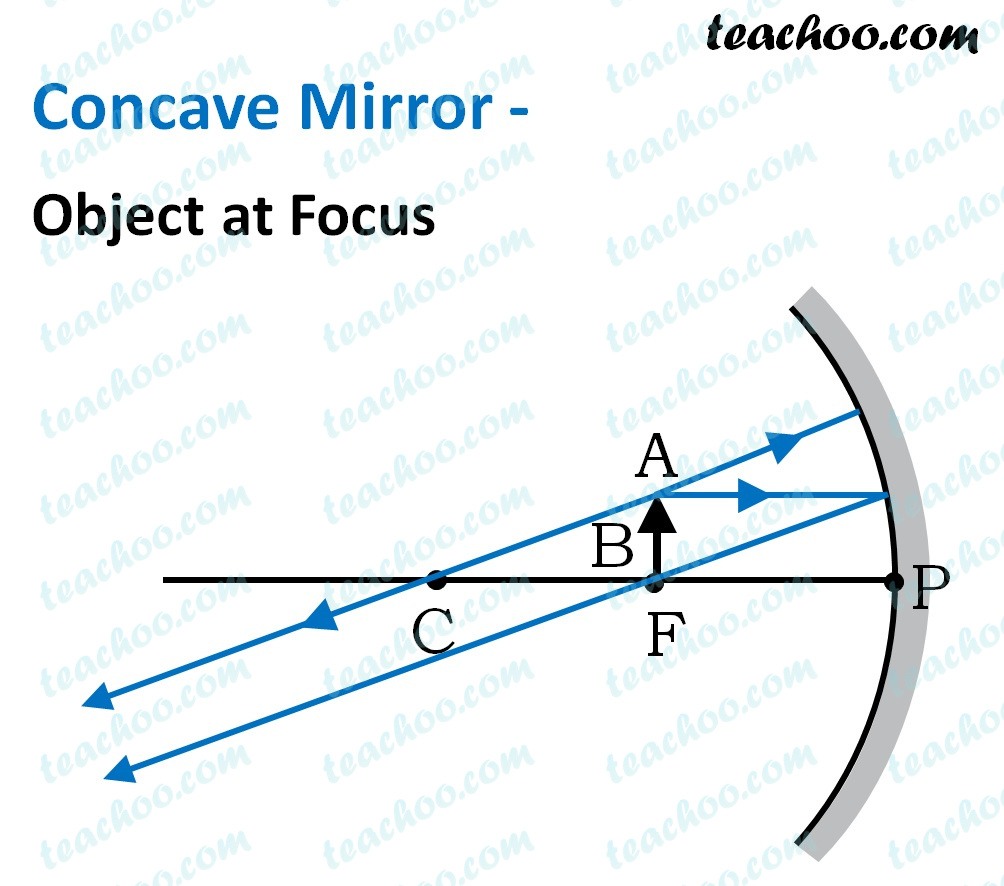Here, Object AB is kept at F

First, we draw a ray parallel to principal axis

So, it passes through focus after reflection

We draw another ray which passes through Center of Curvature

So, the ray will go back along the same path after reflection

We observe that both rays are parallel to each other

Hence we can say that they meet at infinity.

Hence, the rays form an image at infinity

And image formed would be larger than the object

We can say that

• Image is in Front of the Mirror (Real Image)
• Image is Inverted
• Image is larger than the object (Magnified)

### Case 6 - Object is between Pole (P) and Focus (F)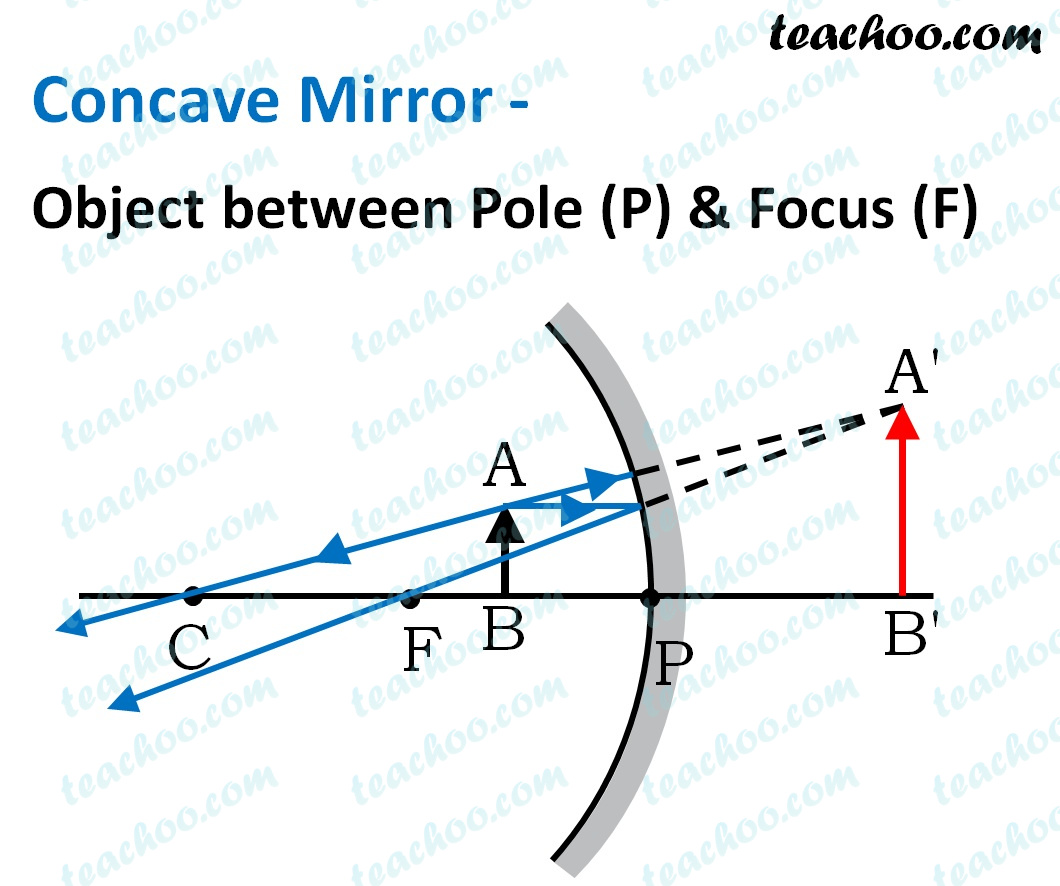Here, Object AB is kept between P and F

First, we draw a ray parallel to principal axis

So, it passes through focus after reflection

We draw another ray which passes through Center of Curvature

So, the ray will go back along the same path after reflection

We observe that both reflected rays are diverging

It means that they would have met at some point

Hence, we extend both reflected rays behind the mirror

We see that the rays form an image behind the mirror.

So, the image is virtual

And image formed would be larger than the object

We can say that

• Image is behind the Mirror (Virtual Image)
• Image is erect
• Image is larger than the object (Magnified)

Note: -
Real image is formed in front of mirror

Virtual Image is formed behind the mirror

And,

Inverted image is upside down

Erect image is straight

## To summarize

 Position of the object Position of the image Size of the image Nature of the image At infinity At the focus F Highly diminished, point-sized Real and inverted Beyond C Between F and C Diminished Real and inverted At C At C Same size Real and inverted Between C and F Beyond C Enlarged Real and inverted At F At infinity Highly enlarged Real and inverted Between P and F Behind the mirror Enlarged Virtual and erect

## Questions

Question 3 Page 168 - Name a mirror that can give an erect and enlarged image of an object

NCERT Question 2 - The image formed by a concave mirror is observed to be virtual, erect and larger than the object. Where should be the position of the object?

(a) Between the principal focus and the centre of curvature

(b) At the centre of curvature

(c) Beyond the centre of curvature

(d) Between the pole of the mirror and its principal focus.

1. Class 10
2. Chapter 10 Class 10 - Light - Reflection and Refraction (Term 1)
3. Concepts